# SUMIFS in external files or two dimensions?

I hope you are also a fan of SUMIFS… this great function can be extremely useful for adding up sub-sets of data. But it has two limitations:

1. Performing calculations on data in other files is not best practice, but it can be a practical solution in some cases. In such cases, a SUMIFS formula can generate errors if the other file is closed.
2. You cannot have criteria in two dimensions (rows AND columns), just one or the other.

There is a way to solve both these issues using the function SUMPRODUCT.

1. SUMPRODUCT in one dimension

Here is an example using small table of data.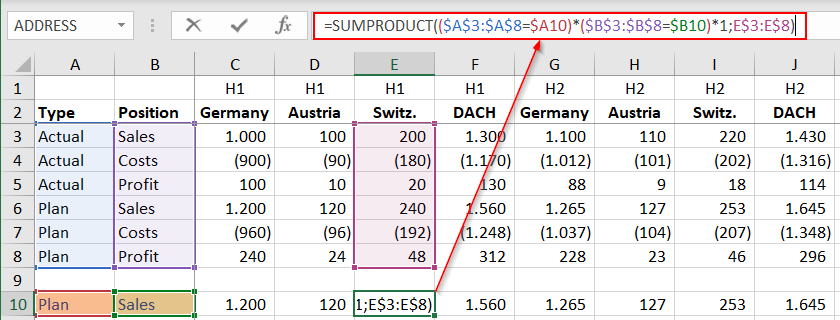As you can see, the formula is like the equivalent SUMIFS formula. Here, however, each criteria range is not separated from its related criteria by a separator (typically a comma or semicolon, depending on your regional settings). Instead, they are separated by an equals sign, which I find easier to read. Each (criteria range = criteria) test is surrounded by brackets and multiplied with the next (criteria range = criteria) test. At the end, you must multiply by one to convert all the true and false results into 1s and 0s and lastly you multiply by the data range which is to be summed. With SUMIF (1 criterion), this data range must be the first argument in the brackets, with SUMIFS (1+ criteria), it must be the last. With SUMPRODUCT, it can be either first or last.

External files

All the function arguments (the bits in the brackets) can refer to an external file.

how2tip: I find it easier to write and test such a formula in the file with the source data and then cut and paste it to the desired location. This is easier because you can (a) better see what you are referring to and (b) the path and file name do not appear in the formula until you cut and paste it, so it is easier to write, understand and test.

2. SUMPRODUCT in two dimensions

The above principles can now be extended to two dimensions, as shown here, using the same data table.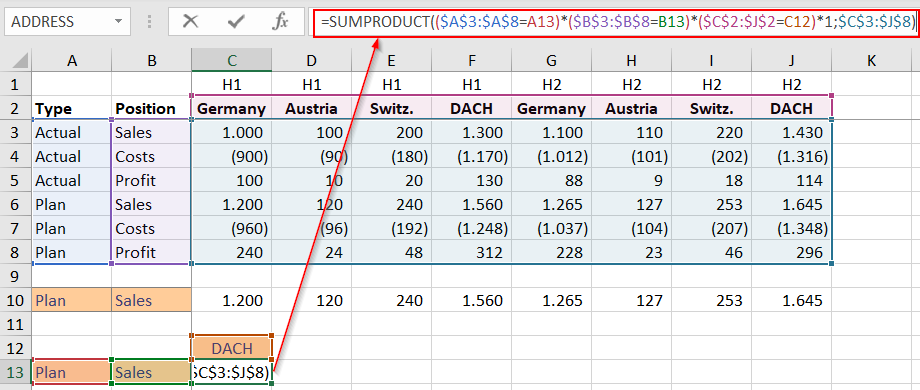Here it is important that (similar to the one dimension example) the criteria ranges match the height or width of the data table, as you can see in the F2 (“edit mode”) screenshot above.

3. Conditional formatting

To put the icing on the cake, I decided to use conditional formatting to automatically highlight (in green) the data cells being added up. These vary, depending upon what criteria are selected in the salmon-coloured input cells.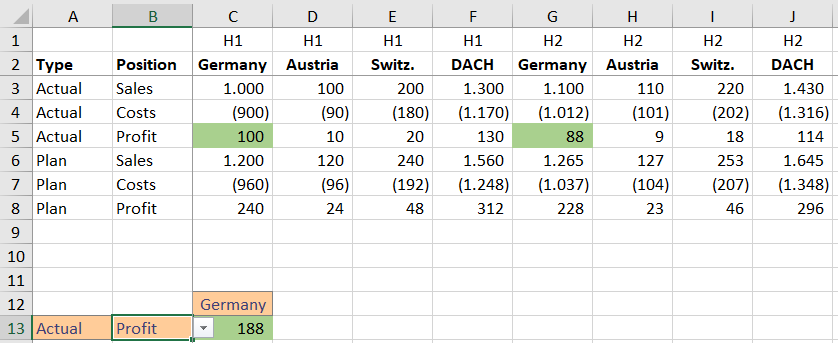The conditional format must be applied to the all data cells in the table since any of them could fulfil the selected conditions.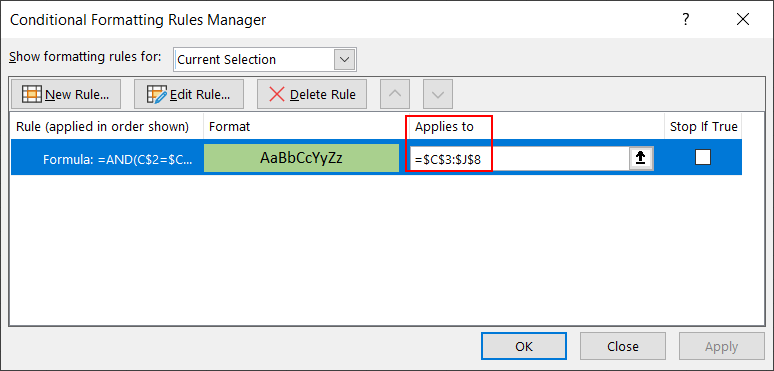The format must highlight cells (in this case in green) if all conditions in the SUMPRODUCT formula are fulfilled, as shown below. Here it is important to pay attention to the dollar (fixing) symbols. Always write the formula from the viewpoint of the cell in the upper left corner of the data range, here C3. The formula must start with an equals sign (as in all Excel formulas) und must give a true or false result. It is the equivalent of writing an IF formula, but you do not need the IF function. Just write the logical test, using “OR” or “AND” as required.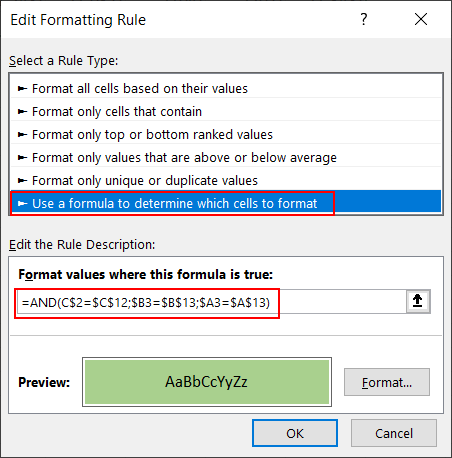That’s it, have fun!